# Casimir element

(diff) ← Older revision | Latest revision (diff) | Newer revision → (diff)

Casimir operator

A central element of special form in the universal enveloping algebra of a semi-simple Lie algebra. Such operators were first introduced, for a particular case, by H. Casimir .

Letbe a semi-simple finite-dimensional Lie algebra over a field of characteristic, and letbe an invariant symmetric bilinear form on(that is,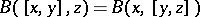for all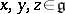) which is non-degenerate on a Cartan subalgebra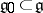. Then a Casimir element of the Lie algebrawith respect to the formis an element of the universal enveloping algebrathat is representable in the form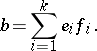Here,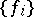are dual bases of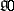with respect to, that is,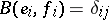,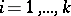, where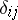is the Kronecker symbol and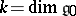. The element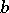does not depend on the choice of the dual bases inand belongs to the centre of. Ifis a simple algebra, then a Casimir element ofdefined by the Killing formis the unique (up to a scalar multiplier) central element in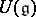that is representable as a homogeneous quadratic polynomial in the elements of.

Every linear representationof a semi-simple algebrain a finite-dimensional spacedefines an invariant symmetric bilinear form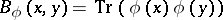on, which is non-degenerate on the subalgebracomplementary to, and therefore also defines some Casimir element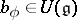. Ifis an irreducible representation, then the extension ofontotakes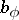into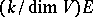.

How to Cite This Entry:
Casimir element. Encyclopedia of Mathematics. URL: http://encyclopediaofmath.org/index.php?title=Casimir_element&oldid=11861
This article was adapted from an original article by D.P. Zhelobenko (originator), which appeared in Encyclopedia of Mathematics - ISBN 1402006098. See original article